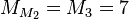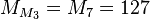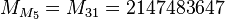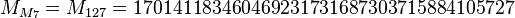Saturday

occurrence 396

Marin Mersenne was a mathematician.  He is mostly known for the Mersenne Primes, but also for the Double Mersenne Primes, which have several 27's.$M_{M_2} = M_3 = 7$$M_{M_3} = M_7 = 127$$M_{M_5} = M_{31} = 2147483647$$M_{M_7} = M_{127} = 170141183460469231731687303715884105727$

He also wrote twenty-seven books on the Geometry of Pierre de la Ramé.

He studied the mathematics of music and invented (I think) the twenty-seven key organ.

Here is another mention of the structure of an organ from a book.